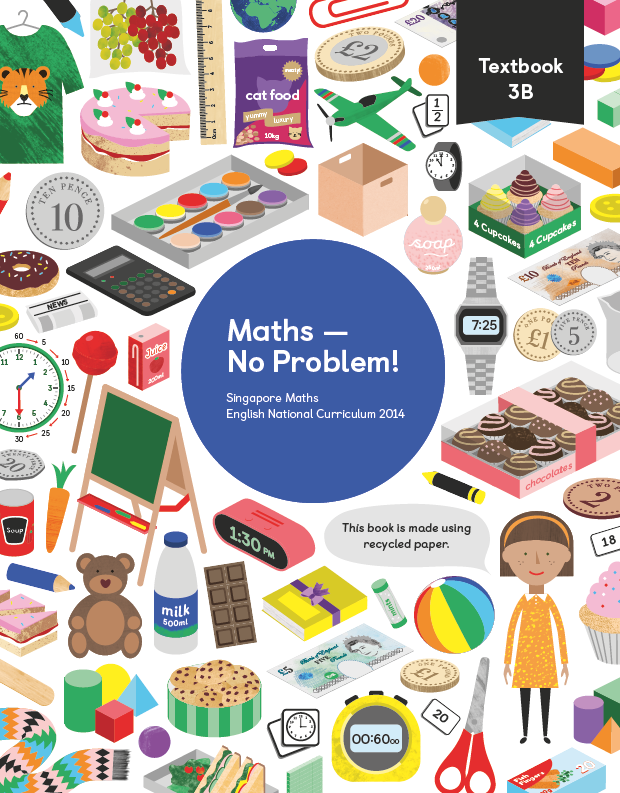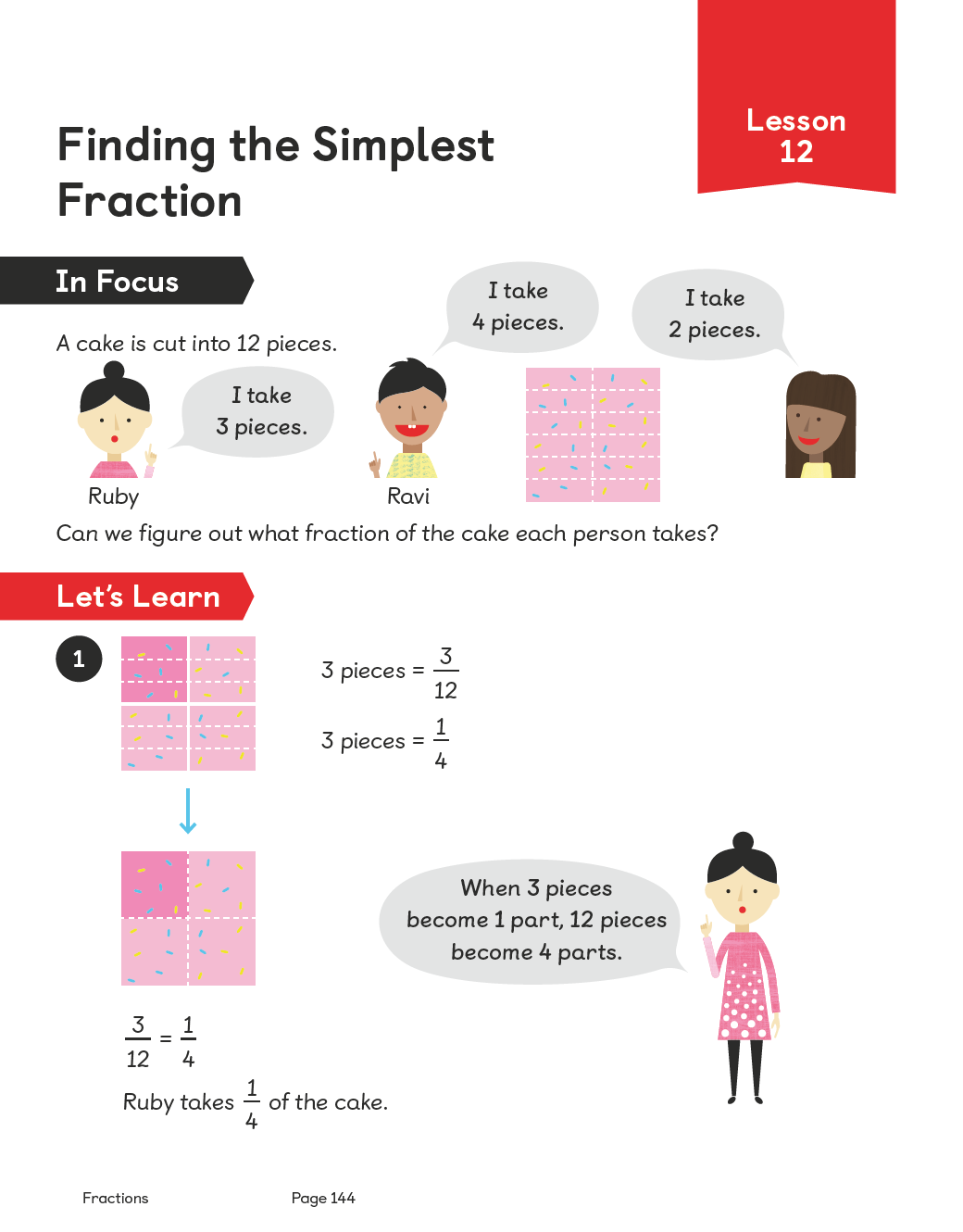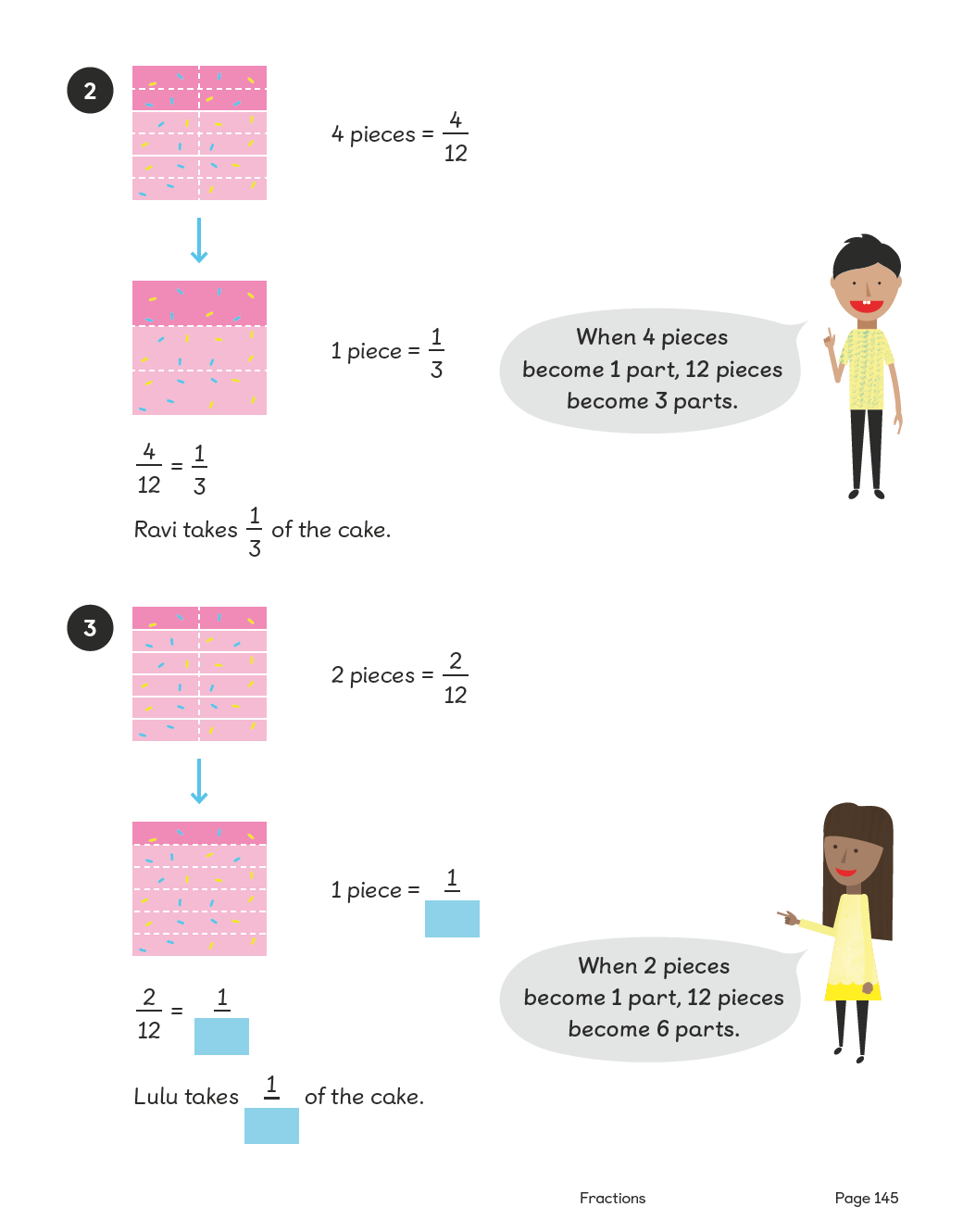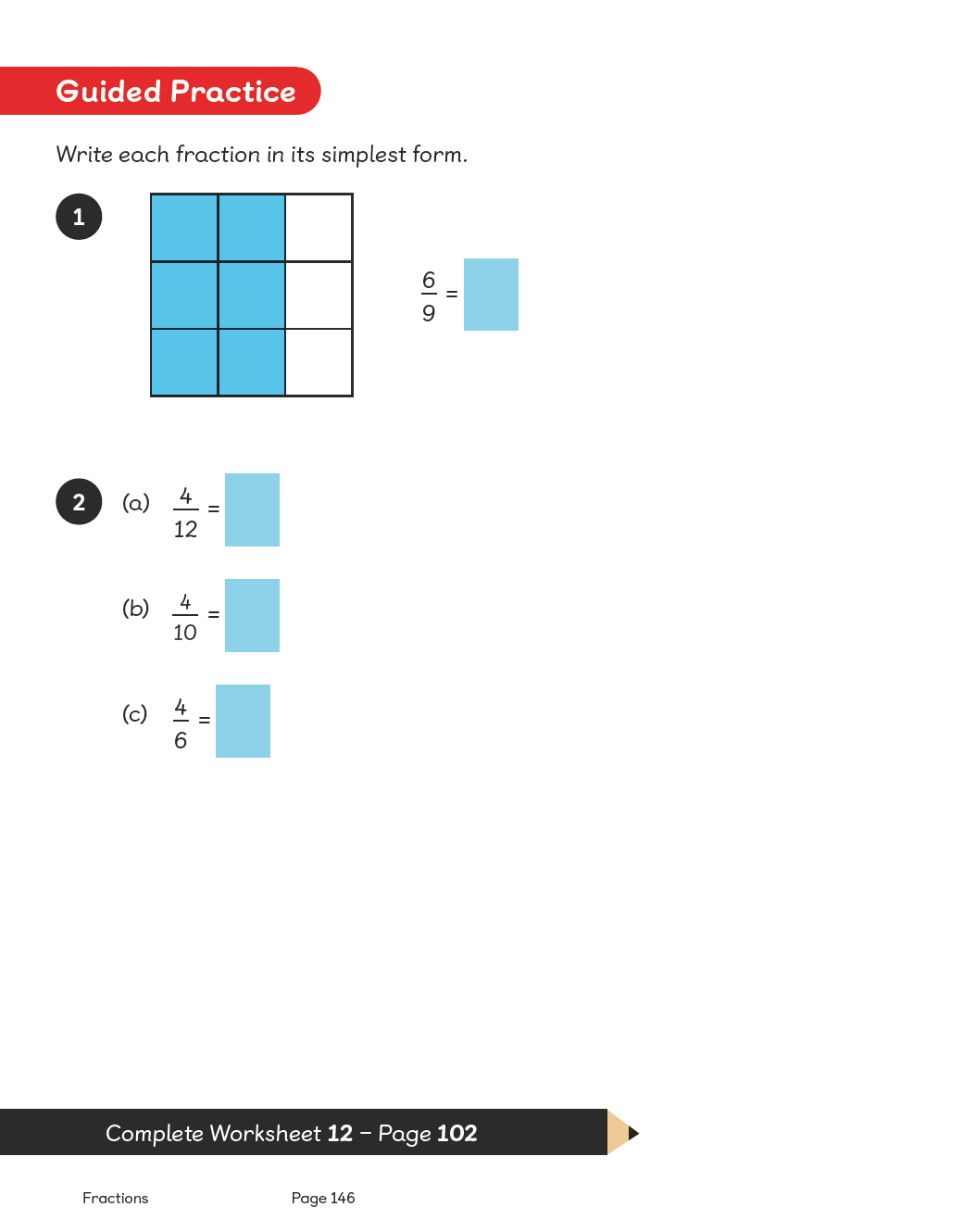Textbook 3B# Maths — No Problem!Textbook 3B

## £9.99

ISBN: 9781910504109
Pages: 268
Availability:
In stock
Qty:

• Lesson 1 - Naming Amounts of Money

To be able to add money by counting on.

• Lesson 2 - Naming Amounts of Money

To be able to add pence to make 1 pound.

• Lesson 3 - Showing Amounts of Money

To be able to add different combinations of coins to make an amount.

• Lesson 4 - Adding Money

To be able to add pounds and pence without renaming.

• Lesson 5 - Adding Money

To be able to add pounds and pence with renaming.

• Lesson 6 - Adding Money

To be able to add pounds and pence with renaming.

• Lesson 7 - Adding Money

To be able to add pounds and pence with renaming.

• Lesson 8 - Subtracting Money

To be able to subtract pounds and pence without renaming.

• Lesson 9 - Subtracting Money

To be able to subtract pounds and pence without renaming.

• Lesson 10 - Subtracting Money

To be able to subtract pounds and pence with renaming.

• Lesson 11 - Subtracting Money

To be able to subtract pounds and pence with renaming.

• Lesson 12 - Calculating Change

To be able to subtract pounds and pence with renaming.

• Lesson 13 - Solving Word Problems

To be able to solve word problems involving addition and subtraction of money.

• Lesson 14 - Solving Word Problems

To be able to solve multi-step word problems involving addition and subtraction of money.

• Lesson 15 - Chapter Consolidation

To be able to use knowledge of money to solve problems.

## Author(s)

Consultant and Author: Dr. Yeap Ban Har
UK Consultant: Dr. Anne Hermanson
Authors: Dr. Foong Pui Yee, Lim Li Gek Pearlyn, Wong Oon Hua